×#### Thank you for registering.

One of our academic counsellors will contact you within 1 working day.

Click to Chat

1800-1023-196

+91-120-4616500

CART 0

• 0

MY CART (5)

Use Coupon: CART20 and get 20% off on all online Study Material

ITEM
DETAILS
MRP
DISCOUNT
FINAL PRICE
Total Price: Rs.

There are no items in this cart.
Continue Shopping• Complete JEE Main/Advanced Course and Test Series
• OFFERED PRICE: Rs. 15,900
• View Details

```Chapter 7: Introduction to Euclid’s Geometry Exercise – 7.1

Question: 1

Define the following terms.

(i)  Line segment

(ii) Collinear points

(iii) Parallel lines

(iv) Intersecting lines

(v) Concurrent lines

(vi) Ray

(vii) Half-line

Solution:

(i) Line-segment:

Give two points A and B on a line I. the connected part (segment) of the line with end points at A and B is called the line segment AB.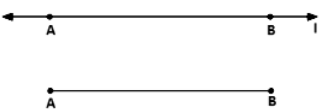(ii) Collinear points:

Three or more points are said to be collinear if there is a line which contains all of them.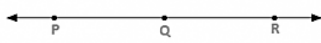(iii) Parallel lines:

Two lines l and m in a plane are said to be parallel lines if they do not intersect each other.(iv) Intersecting lines:

Two lines are intersecting if they have a common point. The common point is called point of intersection.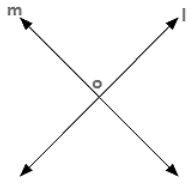(v) Concurrent lines:

Three or more lines are said to be concurrent if there is a point which lies on all of them.(vi) Ray:

A line in which one end point is fixed and the other part can be extended endlessly.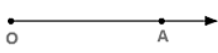(vii) Half-line:

If A, B. C be the points on a line l, such that A lies between B and C, and we delete the point A from line l, the two parts of l that remain are each called half-line.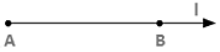Question: 2

(i) How many lines can pan through a given point?

(ii) In how many points can two distinct lines at the most intersect?

Solution:

(i) Infinitely many

(ii) One

Question: 3

(i) Given two points P and Q. Find how many line segments do they determine.

(ii) Name the line segments determined by the three collinear points P. Q and R.

Solution:

(i) One

(ii) PQ, QR, PR

Question: 4

Write the truth value (T/F) of each of the following statements:

(i) Two lines intersect in a point.

(ii) Two lines may intersect in two points

(iii) A segment has no length.

(iv) Two distinct points always determine a line.

(v) Every ray has a finite length.

(vi) A ray has one end-point only.

(vii) A segment has one end-point only.

(viii) The ray AB is same as ray BA.

(ix) Only a single line may pass through a given point.

(x) Two lines are coincident if they have only one point in common

Solution:

(i) False

(ii) False

(iii) False

(iv) True

(v) False

(vi) True

(vii) False

(viii) False

(ix) False

(x) False

Question: 5

In the below figure. Name the following: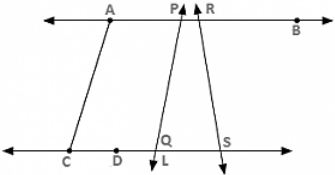Solution:

(i) Five line segments AB, CD, AC, PQ. DS

(ii) Five rays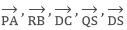(iii) Four collinear points. C, D, Q, S

(iv) Two pairs of non--intersecting line segments AB and CD, AB and LS.

Question: 6

Fill in the blanks so as to make the following statements true:

(i) Two distinct points in a plane determine a _____________ line.

(ii) Two distinct ___________ in a plane cannot have more than one point in common.

(iii) Given a line and a point, not on the line, there is one and only _____________ line which passes through the given point and is _______________ to the given line.

(iv) A line separates a plane into _________ parts namely the __________ and the _____ itself.

Solution:

(i) Unique

(ii) Lines

(iii) Perpendicular, perpendicular

(iv) Three, two half planes, line.
```### Course Features

• 728 Video Lectures
• Revision Notes
• Previous Year Papers
• Mind Map
• Study Planner
• NCERT Solutions
• Discussion Forum
• Test paper with Video Solution